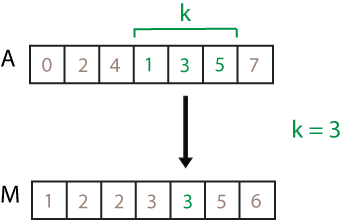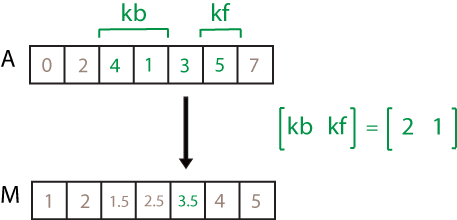# movmedian

## 语法

``M = movmedian(A,k)``
``M = movmedian(A,[kb kf])``
``M = movmedian(___,dim)``
``M = movmedian(___,nanflag)``
``M = movmedian(___,Name,Value)``

## 说明

``M = movmedian(A,k)` 返回由局部 `k` 个数据点的中位数值组成的数组，其中每个中位数基于 `A` 的相邻元素的长度为 `k` 的滑动窗计算得出。当 `k` 为奇数时，窗以当前位置的元素为中心。当 `k` 为偶数时，窗以当前元素及其前一个元素为中心。当没有足够的元素填满窗时，窗将自动在端点处截断。当窗口被截断时，只根据窗口内的元素计算中位数。`M` 与 `A` 的大小相同。如果 `A` 是向量，`movmedian` 将沿向量 `A` 的长度运算。如果 `A` 是多维数组，则 `movmedian` 沿 `A` 的大小不等于 1 的第一个维度进行运算。`

``M = movmedian(A,[kb kf])` 通过长度为 `kb+kf+1` 的窗口计算中位数，其中包括当前位置的元素、前面的 `kb` 个元素和后面的 `kf` 个元素。`

``M = movmedian(___,dim)` 为上述任一语法指定 `A` 的运算维度。例如，如果 `A` 是矩阵，则 `movmedian(A,k,2)` 沿 `A` 的列运算，计算每行的 `k` 个元素的移动中位数。`

``M = movmedian(___,nanflag)` 指定包含还是省略 `A` 中的 `NaN` 值。例如，`movmedian(A,k,"omitnan")` 在计算每个中位数时会忽略 `NaN` 值。默认情况下，`movmedian` 包括 `NaN` 值。`

``M = movmedian(___,Name,Value)` 使用一个或多个名称-值对组参数指定移动中位数的其他参数。例如，如果 `x` 是时间值向量，则 `movmedian(A,k,"SamplePoints",x)` 相对于 `x` 中的时间计算移动中位数。`

## 示例

```A = [4 8 6 -1 -2 -3 -1 3 4 5]; M = movmedian(A,3)```
```M = 1×10 6.0000 6.0000 6.0000 -1.0000 -2.0000 -2.0000 -1.0000 3.0000 4.0000 4.5000 ```

```A = [4 8 6 -1 -2 -3 -1 3 4 5]; M = movmedian(A,[2 0])```
```M = 1×10 4 6 6 6 -1 -2 -2 -1 3 4 ```

`A = [4 8 6; -1 -2 -3; -1 3 4]`
```A = 3×3 4 8 6 -1 -2 -3 -1 3 4 ```
`M = movmedian(A,3,2)`
```M = 3×3 6.0000 6.0000 7.0000 -1.5000 -2.0000 -2.5000 1.0000 3.0000 3.5000 ```

`A = [4 8 NaN -1 -2 -3 NaN 3 4 5];`

`M = movmedian(A,3,"omitnan")`
```M = 1×10 6.0000 6.0000 3.5000 -1.5000 -2.0000 -2.5000 0 3.5000 4.0000 4.5000 ```

```A = [4 8 6 -1 -2 -3]; k = hours(3); t = datetime(2016,1,1,0,0,0) + hours(0:5)```
```t = 1x6 datetime 01-Jan-2016 00:00:00 01-Jan-2016 01:00:00 01-Jan-2016 02:00:00 01-Jan-2016 03:00:00 01-Jan-2016 04:00:00 01-Jan-2016 05:00:00 ```
`M = movmedian(A,k,"SamplePoints",t)`
```M = 1×6 6.0000 6.0000 6.0000 -1.0000 -2.0000 -2.5000 ```

```A = [4 8 6 -1 -2 -3 -1 3 4 5]; M = movmedian(A,3,"Endpoints","discard")```
```M = 1×8 6 6 -1 -2 -2 -1 3 4 ```

## 输入参数• `movmedian(A,k,1)``A` 的每列计算包含 `k` 个元素的移动中位数，并返回一个 `m`×`n` 矩阵。• `movmedian(A,k,2)``A` 的每行计算包含 `k` 个元素的移动中位数，并返回一个 `m`×`n` 矩阵。• `"includemissing"``"includenan"` - 在计算每个中位数时包括 `A` 中的 `NaN` 值。如果窗中的任一元素是 `NaN`，则 `M` 中的对应元素是 `NaN``"includemissing"``"includenan"` 具有相同的行为。

• `"omitmissing"``"omitnan"` - 忽略 `A` 中的所有 `NaN` 值，并基于较少的点计算每个中位数。如果窗中的所有元素都是 `NaN`，则 `M` 中的对应元素是 `NaN``"omitmissing"``"omitnan"` 具有相同的行为。

### 名称-值参数

`"shrink"`在窗接近输入端点时缩小窗口的大小，从而只包括现有元素。
`"discard"`当窗不与现有元素完全重叠时，不输出任何中位数值。
`"fill"`将不存在的元素替换为 `NaN` 值。